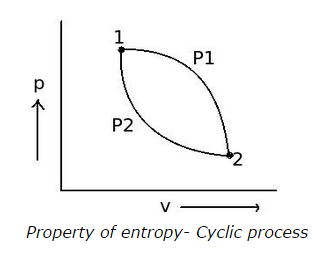# Property of entropy - MCQs with Answers

Q1. When a system is taken from state A to state B through a reversible path 1 and again the system is taken to its initial state A from B through different reversible path 2, then what will be the effect on entropy?

a. entropy increases
b. entropy decreases
c. entropy remains constant
d. none of the above

Q2. Two reversible paths P1 and P2 are represented in the figure and the process represented is a cyclic process. Which will be the correct relation for the cyclic process shown?a. Cyclic ∫P1 P2 (dQ/T) = 0
b. 12 P1 (dQ/T) = 12 P2 (dQ/T)
c. 12 P1 (dQ/T) = 21 P2 (dQ/T)
d. all of the above

ANSWER: d. all of the above

Q3. Which type of function the entropy is?

a. a path function
b. a point function
c. both a. and b.
d. none of the above

Q4. 100 kg of ice at 0 °C is melted completely. What will be the change in entropy in kJ/K, when T2 = 0 °C and latent heat of fusion of ice is taken as 334.774 J/g

a. 0
b. 100.6
c. 122.6
d. 150.6

Q5. The entropy is

a. an intensive property
b. an extensive property
c. both a. and b.
d. none of the above

Q6. How is the entropy change of any irreversible process estimated, when the process is connecting two equilibrium points A and B?

a. by replacing the original process by reversible zigzag path containing reversible adiabatic followed by isothermal and then again reversible adiabatic process between the same equilibrium points A and B

b. by replacing a reversible path between the same equilibrium points A and B

c. both a. and b.

d. none of the above